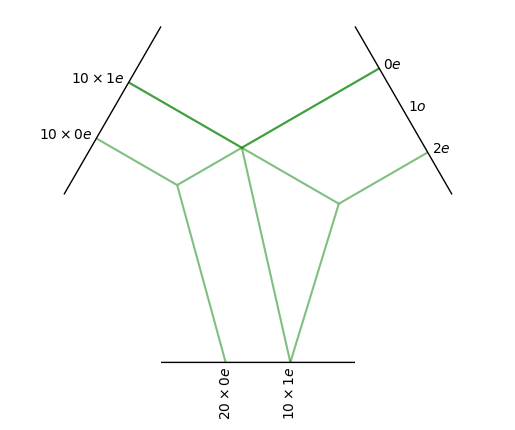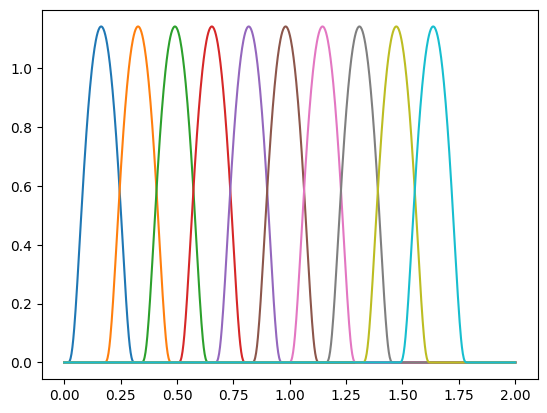# Convolution

In this document we will implement an equivariant convolution with e3nn. We will implement this formula:

$f'_i = \frac{1}{\sqrt{z}} \sum_{j \in \partial(i)} \; f_j \; \otimes\!(h(\|x_{ij}\|)) \; Y(x_{ij} / \|x_{ij}\|)$

where

• $$f_j, f'_i$$ are the nodes input and output

• $$z$$ is the average degree of the nodes

• $$\partial(i)$$ is the set of neighbors of the node $$i$$

• $$x_{ij}$$ is the relative vector

• $$h$$ is a multi layer perceptron

• $$Y$$ is the spherical harmonics

• $$x \; \otimes\!(w) \; y$$ is a tensor product of $$x$$ with $$y$$ parametrized by some weights $$w$$

Boilerplate imports

import torch
from torch_scatter import scatter
from e3nn import o3, nn
from e3nn.math import soft_one_hot_linspace
import matplotlib.pyplot as plt


Let’s first define the irreps of the input and output features.

irreps_input = o3.Irreps("10x0e + 10x1e")
irreps_output = o3.Irreps("20x0e + 10x1e")


And create a random graph using random positions and edges when the relative distance is smaller than max_radius.

# create node positions
num_nodes = 100
pos = torch.randn(num_nodes, 3)  # random node positions

# create edges

print(edge_src.shape)

edge_vec = pos[edge_dst] - pos[edge_src]

# compute z
num_neighbors = len(edge_src) / num_nodes
num_neighbors

torch.Size()

34.92


edge_src and edge_dst contain the indices of the nodes for each edge. And we can also create some random input features.

f_in = irreps_input.randn(num_nodes, -1)


Note that out data is generated with a normal distribution. We will take care of having all the data following the component normalization (see Normalization).

f_in.pow(2).mean()  # should be close to 1

tensor(1.0069)


$Y(x_{ij} / \|x_{ij}\|)$
irreps_sh = o3.Irreps.spherical_harmonics(lmax=2)
print(irreps_sh)

sh = o3.spherical_harmonics(irreps_sh, edge_vec, normalize=True, normalization='component')
# normalize=True ensure that x is divided by |x| before computing the sh

sh.pow(2).mean()  # should be close to 1

1x0e+1x1o+1x2e

tensor(1.0000)


Now we need to compute $$\otimes(w)$$ and $$h$$. Let’s create the tensor product first, it will tell us how many weights it needs.

tp = o3.FullyConnectedTensorProduct(irreps_input, irreps_sh, irreps_output, shared_weights=False)

print(f"{tp} needs {tp.weight_numel} weights")

tp.visualize();

FullyConnectedTensorProduct(10x0e+10x1e x 1x0e+1x1o+1x2e -> 20x0e+10x1e | 400 paths | 400 weights) needs 400 weightsin this particual choice of irreps we can see that the l=1 component of the spherical harmonics cannot be used in the tensor product. In this example it’s the equivariance to inversion that prohibit the use of l=1. If we don’t want the equivariance to inversion we can declare all irreps to be even (irreps_sh = Irreps("0e + 1e + 2e")).

To implement $$h$$ that has to map the relative distances to the weights of the tensor product we will embed the distances using a basis function and then feed this embedding to a neural network. Let’s create that embedding. Here is the base functions we will use:

num_basis = 10

x = torch.linspace(0.0, 2.0, 1000)
y = soft_one_hot_linspace(
x,
start=0.0,
number=num_basis,
basis='smooth_finite',
cutoff=True,
)

plt.plot(x, y);Note that this set of functions are all smooth and are strictly zero beyond max_radius. This is useful to get a convolution that is smooth although the sharp cutoff at max_radius.

Let’s use this embedding for the edge distances and normalize it properly (component i.e. second moment close to 1).

edge_length_embedding = soft_one_hot_linspace(
edge_vec.norm(dim=1),
start=0.0,
number=num_basis,
basis='smooth_finite',
cutoff=True,
)
edge_length_embedding = edge_length_embedding.mul(num_basis**0.5)

print(edge_length_embedding.shape)
edge_length_embedding.pow(2).mean()  # the second moment

torch.Size([3492, 10])

tensor(0.9156)


Now we can create a MLP and feed it

fc = nn.FullyConnectedNet([num_basis, 16, tp.weight_numel], torch.relu)
weight = fc(edge_length_embedding)

print(weight.shape)
print(len(edge_src), tp.weight_numel)

# For a proper notmalization, the weights also need to be mean 0
print(weight.mean(), weight.std())  # should close to 0 and 1

torch.Size([3492, 400])
3492 400


Now we can compute the term

$f_j \; \otimes\!(h(\|x_{ij}\|)) \; Y(x_{ij} / \|x_{ij}\|)$

The idea is to compute this quantity per edges, so we will need to “lift” the input feature to the edges. For that we use edge_src that contains, for each edge, the index of the source node.

summand = tp(f_in[edge_src], sh, weight)

print(summand.shape)
print(summand.pow(2).mean())  # should be close to 1

torch.Size([3492, 50])


Only the sum over the neighbors is remaining

$f'_i = \frac{1}{\sqrt{z}} \sum_{j \in \partial(i)} \; f_j \; \otimes\!(h(\|x_{ij}\|)) \; Y(x_{ij} / \|x_{ij}\|)$
f_out = scatter(summand, edge_dst, dim=0, dim_size=num_nodes)

f_out = f_out.div(num_neighbors**0.5)

f_out.pow(2).mean()  # should be close to 1

tensor(0.7333, grad_fn=<MeanBackward0>)


Now we can put everything into a function

def conv(f_in, pos):
edge_vec = pos[edge_dst] - pos[edge_src]
sh = o3.spherical_harmonics(irreps_sh, edge_vec, normalize=True, normalization='component')
emb = soft_one_hot_linspace(edge_vec.norm(dim=1), 0.0, max_radius, num_basis, basis='smooth_finite', cutoff=True).mul(num_basis**0.5)
return scatter(tp(f_in[edge_src], sh, fc(emb)), edge_dst, dim=0, dim_size=num_nodes).div(num_neighbors**0.5)


Now we can check the equivariance

rot = o3.rand_matrix()
D_in = irreps_input.D_from_matrix(rot)
D_out = irreps_output.D_from_matrix(rot)

# rotate before
f_before = conv(f_in @ D_in.T, pos @ rot.T)

# rotate after
f_after = conv(f_in, pos) @ D_out.T

torch.allclose(f_before, f_after, rtol=1e-4, atol=1e-4)

True


The tensor product dominates the execution time:

import time
wall = time.perf_counter()

edge_vec = pos[edge_dst] - pos[edge_src]
print(time.perf_counter() - wall); wall = time.perf_counter()

sh = o3.spherical_harmonics(irreps_sh, edge_vec, normalize=True, normalization='component')
print(time.perf_counter() - wall); wall = time.perf_counter()

emb = soft_one_hot_linspace(edge_vec.norm(dim=1), 0.0, max_radius, num_basis, basis='smooth_finite', cutoff=True).mul(num_basis**0.5)
print(time.perf_counter() - wall); wall = time.perf_counter()

weight = fc(emb)
print(time.perf_counter() - wall); wall = time.perf_counter()

summand = tp(f_in[edge_src], sh, weight)
print(time.perf_counter() - wall); wall = time.perf_counter()

scatter(summand, edge_dst, dim=0, dim_size=num_nodes).div(num_neighbors**0.5)
print(time.perf_counter() - wall); wall = time.perf_counter()

0.0016399270000420074
0.0019641139999748702
0.002849847999868871
0.0012280769999506447
0.005996675999995205
0.0006150109998088737